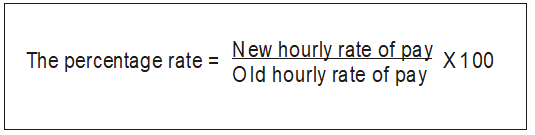Follow

# Calculate Back Pays

To Calculate The Amount Of The Back Pay

1. Calculate the percentage of the increase to calculate the amount of back pay that is owed to the employee.
2. Divide the new pay rate by the old and then multiply this by 100 to get the increase as a percentage.For Example
If Anna is currently paid \$10.50 per hour and the new rate is \$11.75, the calculation will be:Therefore, Anna’s rate has increased by 111.9%. (To check this calculation \$10.50 x 111.9% = \$11.75).
3. Generally, all pay rates (full-time, Saturday, Sunday, overtime etc.) will increase by this percentage, as in most cases every pay rate is relative to the full-time weekday pay rate.
• You may wish to take 2-4 common rates and check them to ensure you come up with the same percentage. You will sometimes find that each pay rate increases by a different percentage.
• Look at the gross over the pay weeks since the pay rise was approved. To do this click, Reports > Employee > Payment Summary, or Reports > Payment Summary, selecting the required dates, for all employees.
• Once you know the total gross, multiply this by the percentage rate of the increase to give you the amount of the back pay.